# Modern Robotics Errata

(Difference between revisions)

Errata below are for the preprint version posted on November 29, 2016.

## Contents

### Chapter 1

• p4: "screw theor." should be "screw theory." (near bottom of page)

### Chapter 3

• p93: The definition of the Trans(p) matrix should have the identity matrix as the top left 3x3 submatrix (shown in the book as the zero matrix).
• p101: The sentence "More generally, for any two frames {c} and {c}..." should be "More generally, for any two frames {c} and {d}..."

### Chapter 11

• p419, the line just before equation (11.16): the expression should read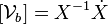$[\mathcal{V}_b] = X^{-1} \dot{X}$ (the square brackets around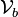$\mathcal{V}_b$ are missing).

### Chapter 12

• p466, 467, 468: Typos in the first equation of the list of three near the bottom of page 466 are propagated to pages 467 and 468. The first equation should be vAx − ωAzpAy + ωAypAz = vBx − ωBzpBy + ωBypBz. This affects the figure at bottom left of Figure 12.3 on p467. Equation (12.10) should be vAx − 2ωAz = vBx − 2ωBz. The first rolling constraint on p468 should then be vAx − 2ωAz = 0.

### Chapter 8

• In the first line of the first (unnumbered) equation of Chapter 8.2.3, the expression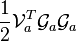$\frac{1}{2} \mathcal{V}_a^T \mathcal{G}_a \mathcal{G}_a$ should be replaced by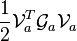$\frac{1}{2} \mathcal{V}_a^T \mathcal{G}_a \mathcal{V}_a$.

### Chapter 13

• p518, equations (13.7) and (13.8): the rows in the matrices should be numbered to m, not n.
• p550, line just after equation (13.37): the expression should read$[\mathcal{V}_d(t)] = X_d^{-1}(t) \dot{X}_d(t)$ (the square brackets around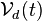$\mathcal{V}_d(t)$ are missing).

### Appendix B

• p577: First line of sec B.1.1, the angle ranges should be$\alpha, \gamma \in (-\pi,\pi]$. Case (b) should be "If r31 = − 1". Case (c) should be "If r31 = 1".